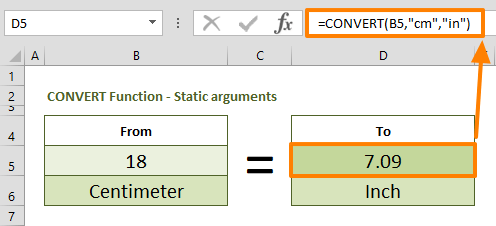Working with multiple units of measure can often become a challenge, going back and forth between various calculators and your Excel workbooks. In this guide, we are going to show you how to convert cm to inches and inches to centimeters using various methods.

## The Manual approach

The first approach we are going to show you is the manual way. You can convert cm to inches with a simple math calculation. 1 inch is approximately equal to 2.54 cm. Thus, you need to divide the centimeter-value by 2.54 to get the value in inches.

Let's say the cell C2 contains a value in centimeters. Simply use a formula like below to find the value in inches.

=C2/2.54In a similar way, you can convert inches to centimeters using the same idea. The example above shows that multiplying an inch-value by 2.54 returns the value in centimeters. If we assume that the cell C5 contains the inch value, the conversion formula will be,

=C5/2.54

Now, let's take a look at a more dynamic (and relatively easy to remember) approach.

## Using the CONVERT Function

Another way to convert cm to inches is using the CONVERT function. The CONVERT function can convert a number from one measurement system to another. The best thing about this approach is that the function is not limited to cm and inches units only.  You can convert weight, distance, temperature, and other units between each other.

Briefly, you need to enter the value for both measurement types into the function. Excel also accepts string abbreviations of the measurement types:

• Centimeters: "cm"
• Inches: "in"

As a result, to convert the value in cell B5, you need to use a formula like below:

=CONVERT(B5,"cm","in")To make the conversion in the opposite direction, simply replace the measurement arguments. The following formula converts the value from inches to cm.

=CONVERT(B5,"in","cm")